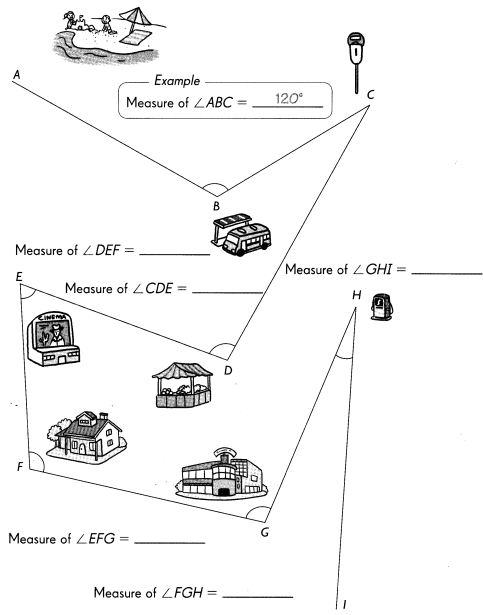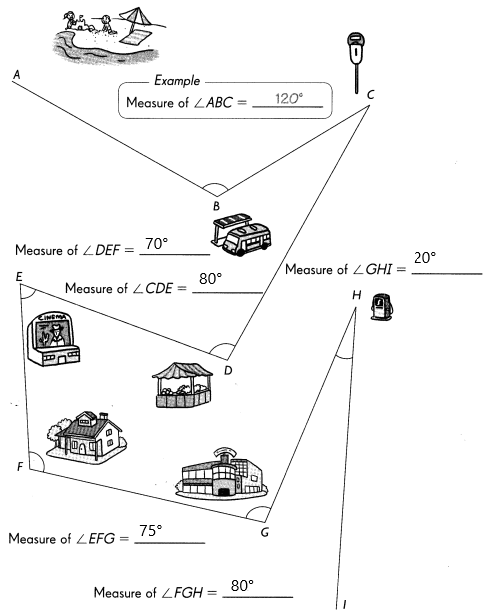# Math in Focus Grade 4 Chapter 9 Practice 1 Answer Key Understanding and Measuring Angles

Practice the problems of Math in Focus Grade 4 Workbook Answer Key Chapter 9 Practice 1 Understanding and Measuring Angles to score better marks in the exam.

## Math in Focus Grade 4 Chapter 9 Practice 1 Answer Key Understanding and Measuring Angles

Name the angles in two ways.

Example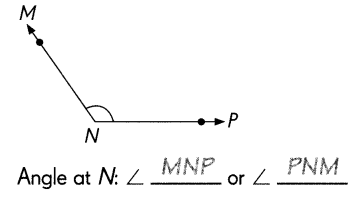Question 1.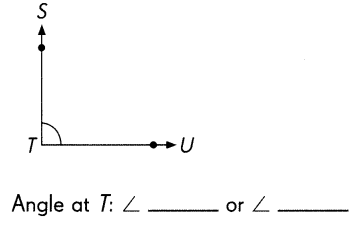∠STU or ∠UTS.

Explanation:
The name of the angle T is ∠STU or ∠UTS.

Question 2.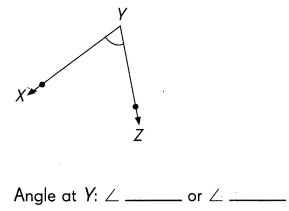∠XYZ or ∠ZYX.

Explanation:
The name of the angle Y is ∠XYZ or ∠ZYX.

Question 3.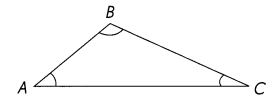Angle at A: ∠___ or ∠___
Angle at B: ∠___ or ∠___
Angle at C: ∠___ or ∠___
∠BAC or ∠CAB,
∠ABC or ∠CBA,
∠BCA or ∠ACB.

Explanation:
The name of angle A is ∠BAC or ∠CAB.
The name of angle B is ∠ABC or ∠CBA.
The name of angle A is ∠BCA or ∠ACB.

Name the marked angles in two ways.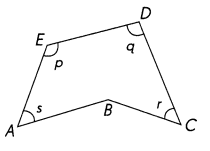Example
∠p: ∠AED or ∠DEA

Question 4.
∠q: ∠____ or ∠_____
∠q: ∠EDC or ∠CDE

Explanation:
The marked angle ∠q is ∠EDC or ∠CDE.

Question 5.
∠r: ∠____ or ∠_____
∠r: ∠BCD or ∠DCB.

Explanation:
The marked angle ∠r is ∠BCD or ∠DCB.

Question 6.
∠s: ∠____ or ∠_____
∠s: ∠BAE or ∠EAB.

Explanation:
The marked angle ∠s is ∠BAE or ∠EAB.

Name the marked angles in two ways.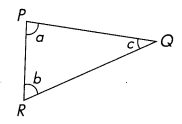Question 7.
∠PRQ: ∠___ or ∠___
∠PRQ: ∠b or ∠QRP.

Explanation:
The name of the marked ∠PRQ is ∠b or ∠QRP.

Question 8.
∠QPR: ∠___ or ∠___
∠QPR: ∠a or ∠RPQ.

Explanation:
The name of the marked ∠QPR is ∠a or ∠RPQ.

Decide which scale you would use to measure each angle.
Fill in the blanks with inner scale or outer scale.

Examples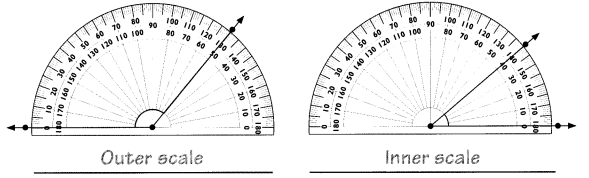Question 9.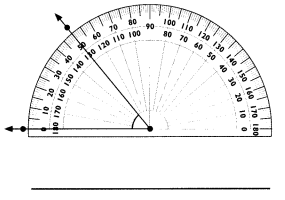Outer scale.

Explanation:
Here, the angle opens to the left of the protractor, so the shown image is an outer scale.

Question 10.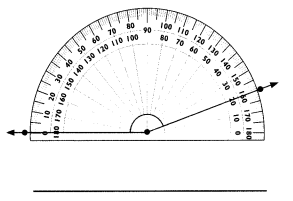Outer scale.

Explanation:
Here, the angle opens to the left of the protractor, so the shown image is an outer scale.

Question 11.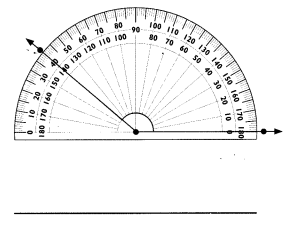Inner scale.

Explanation:
Here, the angle opens to the right of the protractor, so the shown image is an inner scale.

Question 12.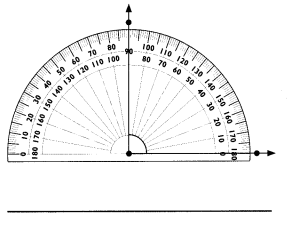Inner scale.

Explanation:
Here, the angle opens to the left of the protractor, so the shown image is an inner scale.

Peter is walking along a path. Measure the marked angles along this path.

Question 17.